Latest Episode
Play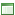Keith and The Girl Forums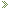Keith and The Girl ForumsShow Talk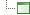1281: 10001110101 IN 3-D
 Register FAQ Calendar Mark Forums Read

 Show Talk Talk about the show

 View Poll Results: Is human praise meaningless if we are not following God's directives? Yes19 13.57% No121 86.43% Voters: 140. You may not vote on this poll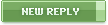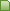10-29-2010, 03:48 AM #11 (permalink) Senior Member   Join Date: Mar 2009 Location: Northern Italy (No Guidos Here) Posts: 6,781 FY:SY=P!:SP! (Offline)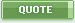10-29-2010, 06:50 AM #12 (permalink) Senior Member   Join Date: Nov 2008 Location: Aldergrove, B.C. Posts: 170 30/Female/Aldergrove, B.C./ no....Cup size C Last edited by funnygurl1979; 10-29-2010 at 06:51 AM. Reason: Forgot cup size (Offline)10-29-2010, 07:00 AM   #13 (permalink)
Senior Member

Join Date: Jun 2009
Posts: 1,122
Quote:
 I think A/S/L should include F You guys fucking someone?
No, I'm too busy fucking your mind.
(Offline)10-29-2010, 09:19 AM   #14 (permalink)
Senior Member

Join Date: Aug 2008
Posts: 991
Quote:
 Originally Posted by DWarrior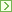Yay, my kind of title! Here's a little of foundations to extend your computer science knowledge: The reason 0s and 1s are used is because, as you said, they can model "off" and "on" (0 for nothing, 1 for something), and it's a lot easier to make a two-state switch than a 3-state switch. The most basic motivation for creating computers is to...compute stuff, and since it's also congruent with my interests, I know a bit of that. Anything more advanced, I'll leave to the computer nerds: All numbers can be represented as binary sequences, so for whole numbers the easiest way is to make each place a power of 2, remember that 2^0 (2 to 0th power) is 1. So 0 is still 0, 1 is still 1, but now 10 is 2 (2*1 + 1*0), 110 is 6 (4*1+2*1+1*0), 1111 is 15 (8*1+4*1+2*1+1*1). You can do all math in binary, it's just a bit strange, and nobody sane remembers a multiplication table in binary. But if you want to add 5+3, in binary it's 101+11=1000. 10-7 is 1010-111=11. Multiplying by 2 is easy as multiplying by 10 in binary: 7*2 is 111*10=1110 And you also have propositional logic, let False = 0, True = 1. A logical variable A takes on only one of two possible values, 0 (for False) or 1 (for True). Define three operations: Given some value A, define ~A (read "not A") as its opposite. So ~0=1, ~1=0. Given A and B, define (A v B) (read "A or B") as 1 if at least one of them is 1. So (0 v 0)=0, but all other cases are 1. Given A and B, define (A ^ B) (read "A and B") as 1 if both of them are 1s, otherwise 0. So (1 ^ 1)=1, everything else is 0. If you imagine A and B as some sort of pipes with water (or wires with electricity), you can construct "logic gates" that will produce flow corresponding to the desired operation. For example, an "OR gate" with input tubes A and B is simply the merging of the two tubes. If water flows through one of them, water will flow out, otherwise nothing. A "NOT gate" with input A would need a separate stream that would flow out if nothing flows out of A or would get deflected if there's a stream at A. Of course, it's much more convenient to manipulate electronic logic gates than pipes with water. This is usually done on a circuit board. We now have the tools to make a very basic adding calculator. Here's one that adds two numbers up to 7: Given two numbers, A and B, write them in binary and each up to three binary digits long (so up to 7), write A as (a3 a2 a1) and B as (b3 b2 b1), where a1 is the first digit of A, a2 is the second digit, and so on. If the numbers are less than 3 digits long, then just tack on 0s on the left (so if A=1, write 001). A+B=C, where C can be up to four digits long, written as (c4 c3 c2 c1). Then the propositional logic formulas for addition are: c1 = (a1 v b1) ^ ~(a1 ^ b1) c2 = (((a1 ^ b1) v ((a2 v b2) ^ ~(a2 ^ b2))) ^ ~((a1 ^ b1) ^ ((a2 v b2) ^ ~(a2 ^ b2)))) c3 = (((((a2 ^ b2) v (a2 ^ (a1 ^ b1))) v (b2 ^ (a1 ^ b1))) v ((a3 v b3) ^ ~(a3 ^ b3))) ^ ~((((a2 ^ b2) v (a2 ^ (a1 ^ b1))) v (b2 ^ (a1 ^ b1))) ^ ((a3 v b3) ^ ~(a3 ^ b3)))) c4 = (((a3 ^ b3) v (a3 ^ (((a2 ^ b2) v (a2 ^ (a1 ^ b1))) v (b2 ^ (a1 ^ b1))))) v (b3 ^ (((a2 ^ b2) v (a2 ^ (a1 ^ b1))) v (b2 ^ (a1 ^ b1))))) If you want to add 2+6, input for A (0, 1, 0) and for B (1, 1, 0), and if you go through the equations, you will see C comes out to be (1, 0, 0, 0), which is just 8 in binary. If you had a circuit board and a bunch of logic gates at your disposal, and batteries/lightbulbs for input and output, you could construct this calculator. In practice, these gates are constructed on a molecular scale, so you have millions of them packed in one chip.(Offline)10-29-2010, 09:49 AM #15 (permalink) Member   Join Date: Oct 2010 Location: Missouri Posts: 97 I know a little code... My interest in anything a certain douche says? - 000000000000 How do you write out "USA" in code? - #111111111goldmedal How big a douche DWarrior is? - <#\$stretchingtoinfinit y%> ASL Keith! Yeah buddy whooo! (Offline)10-29-2010, 10:06 AM   #16 (permalink)
Senior Member

Join Date: Nov 2008
Location: Arizona
Posts: 641
Quote:
 36/Male/NYC/Yes
But, would you be able to say yes if people didn't figure out that kind of stuff all those years ago? Just sayin', you can't have Skype Fucking without someone figuring out the computer science, and a whole bunch of other people learning it so they can maintain and grow upon it.
(Offline)10-29-2010, 10:39 AM #17 (permalink) Junior Member   Join Date: Oct 2010 Posts: 4 This is all well and good but why isn't anyone talking about MEEEEEEEEEEEEE!!!!! or should I say 11100100100101010000101110101!!!!!! (Offline)10-29-2010, 11:01 AM   #18 (permalink)
Member

Join Date: Oct 2010
Location: Dallas Via Detroitish
Posts: 72
Quote:
 This is all well and good but why isn't anyone talking about MEEEEEEEEEEEEE!!!!! or should I say 11100100100101010000101110101!!!!!!
Fuuucckkk yoouuuuu and welcome to the forums.

I got my eye on you robot.
(Offline)10-29-2010, 11:26 AM #19 (permalink) Junior Member   Join Date: Oct 2010 Posts: 4 Ok, that's good enough for me. Thanks Doug. (Offline)10-29-2010, 11:36 AM   #20 (permalink)
Senior Member

Join Date: May 2010
Location: West Virginia
Posts: 105
Quote:
 Originally Posted by JunkensteinFY:SY=P!:SP!
Gah, you're a math whiz as well? How'd I ever get so lucky?
(Offline)Currently Active Users Viewing This Thread: 1 (0 members and 1 guests)
 Thread Tools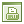Show Printable Version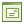Email this Page Display Modes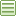Linear Mode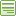Switch to Hybrid Mode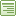Switch to Threaded Mode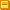Posting Rules You may not post new threads You may not post replies You may not post attachments You may not edit your posts BB code is On Smilies are On [IMG] code is On HTML code is OffTrackbacks are On Pingbacks are On Refbacks are Off Forum Rules

All times are GMT -5. The time now is 02:02 AM.

 Keith and The Girl - TopKeith and The GirlAd Management plugin by RedTyger